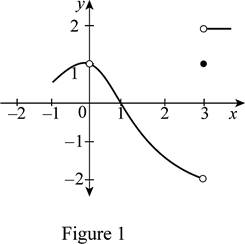# The graph of f ( x ) which satisfies the conditions, lim x → 0 f ( x ) = 1 , lim x → 3 − f ( x ) = − 2 , lim x → 3 + f ( x ) = 2 , f ( 0 ) = − 1 and f ( 3 ) = 1 .### Single Variable Calculus: Concepts...

4th Edition
James Stewart
Publisher: Cengage Learning
ISBN: 9781337687805### Single Variable Calculus: Concepts...

4th Edition
James Stewart
Publisher: Cengage Learning
ISBN: 9781337687805

#### Solutions

Chapter 2.2, Problem 14E
To determine

## To sketch: The graph of f(x) which satisfies the conditions, limx→0f(x)=1,limx→3−f(x)=−2,limx→3+f(x)=2, f(0)=−1 and f(3)=1.

Expert Solution

### Explanation of Solution

Graph:

Given that, limx0f(x)=1,limx3f(x)=2,limx3+f(x)=2, f(0)=1 and f(3)=1.

Here, the left hand limit and the right hand limit of limx3f(x) are not the same.

Hence, limx3f(x) does not exist.

Moreover, according to the given conditions the graph must contain two points such that (0, −1) as f(0)=1  and (3, 1) as f(3)=1.

Thus, the possible graph of f(x) is shown below in Figure 1.In Figure 1, open dots indicate that the points are excluded.

### Have a homework question?

Subscribe to bartleby learn! Ask subject matter experts 30 homework questions each month. Plus, you’ll have access to millions of step-by-step textbook answers!